# DS with JS — Linked Lists — II

## Data Structures with JavaScript — Chapter Two — Doubly Linked Lists

A doubly linked list is a linked data structure that consists of a set of sequentially linked records called nodes. Each node contains two fields, called links, that are references to the previous and to the next node in the sequence of nodes.
⚠️ If you wan’t to learn more about linked-lists click here

#### Before Proceeding further…

This post is continued from DS with JS — Linked Lists, If you haven’t gone through that yet, make sure you do before proceeding further…

### ⚜️ The list

1. Append
2. AppendAt
3. Remove
4. RemoveAt
5. Reverse
6. Swap
7. IsEmpty & Length
8. Traverse
9. Display
10. Search

### Lets’ begin!!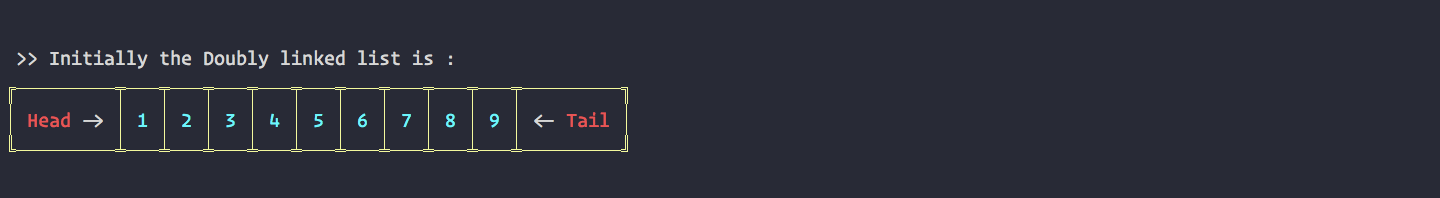Initial List
All the code snippets you will see below are just Pseudo code. If you want to see working code — click here

#### 🔅 Append

`append( item ) {  let node = new Node( item );`
`  if(!this.head) {    this.head = node;    this.tail = node;  } else {    node.prev = this.tail;    this.tail.next = node;    this.tail = node  }}`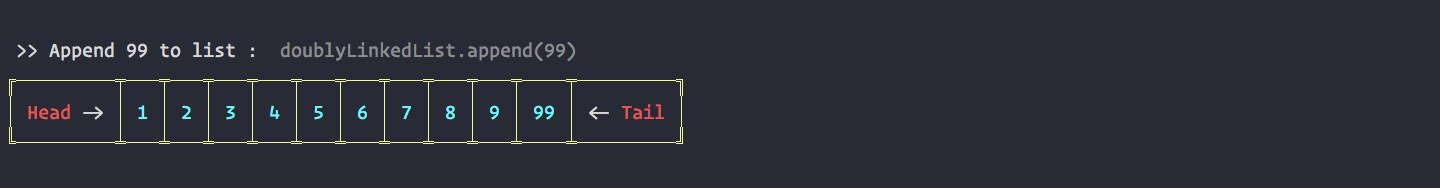Append Operation

#### 🔅 AppendAt

`appendAt( pos, item ) {   let current = this.head;   let counter = 1;   let node = new Node( item );   if( pos == 0 ) {     this.head.prev = node     node.next = this.head     this.head = node   } else {     while(current) {      current = current.next;      if( counter == pos ) {        node.prev = current.prev        current.prev.next = node        node.next = current        current.prev = node      }      counter++    }  }}`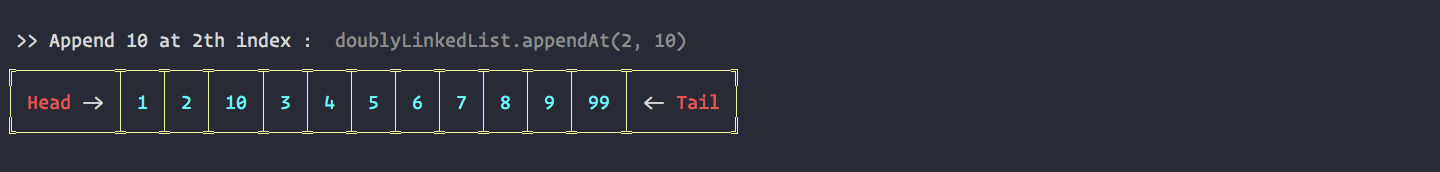AppendAt Operation

#### 🔅 Remove

`remove( item ) {  let current = this.head;  while( current ) {    if( current.data === item ) {      if( current == this.head && current == this.tail ) {        this.head = null;        this.tail = null;      } else if ( current == this.head ) {        this.head = this.head.next        this.head.prev = null      } else if ( current == this.tail ) {        this.tail = this.tail.prev;        this.tail.next = null;      } else {        current.prev.next = current.next;        current.next.prev = current.prev;      }   }   current = current.next  }}`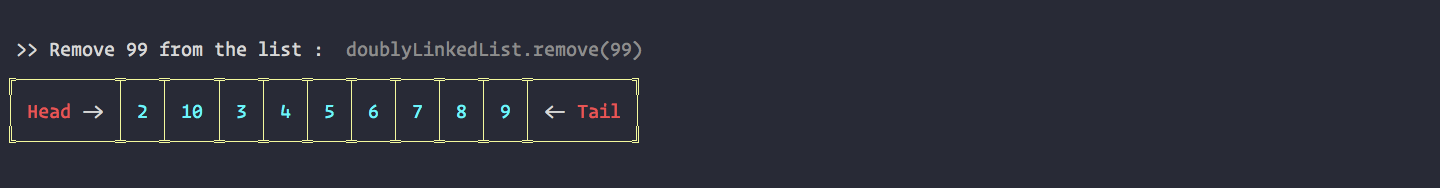Remove Operation

#### 🔅 RemoveAt

`removeAt( pos ) {  let current = this.head;  let counter = 1;  if( pos == 0 ) {   this.head = this.head.next;   this.head.prev = null;  } else {   while( current ) {    current = current.next    if ( current == this.tail ) {     this.tail = this.tail.prev;     this.tail.next = null;    } else if( counter == pos ) {     current.prev.next = current.next;     current.next.prev = current.prev;     break;    }    counter++;   }  }}`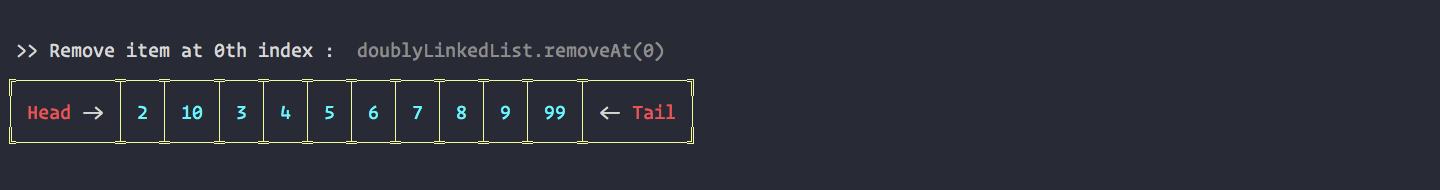RemoveAt Operation

#### 🔅 Reverse

`reverse(){  let current = this.head;  let prev = null;  while( current ){   let next = current.next   current.next = prev   current.prev = next   prev = current   current = next  }  this.tail = this.head  this.head = prev}`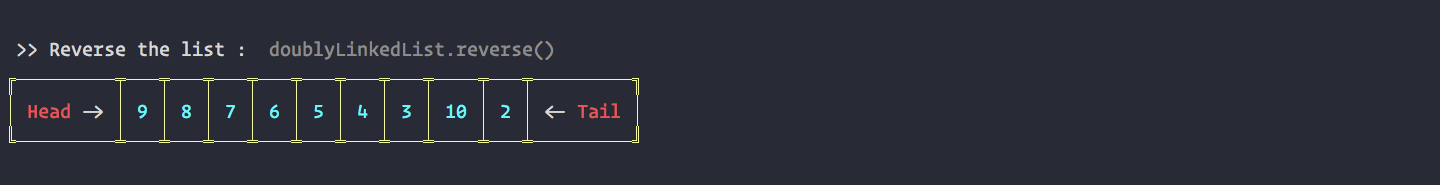Reverse Operation

#### 🔅 Swap

`swap( nodeOne, nodeTwo ) {   let current = this.head;   let counter = 0;   let firstNode;   while( current !== null ) {     if( counter == nodeOne ){       firstNode = current;     } else if( counter == nodeTwo ) {       let temp = current.data;       current.data = firstNode.data;       firstNode.data = temp;     }     current = current.next;     counter++;   }   return true}`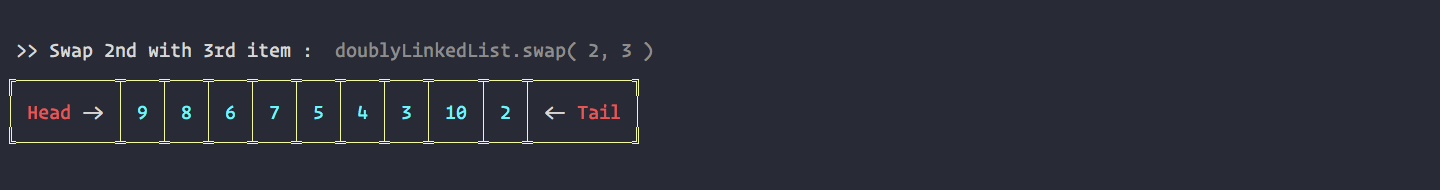Swap Operation

#### 🔅 IsEmpty & Length

`length() {  let current = this.head;  let counter = 0;  while( current !== null ) {   counter++   current = current.next  }  return counter;}`
`isEmpty() {  return this.length() < 1}`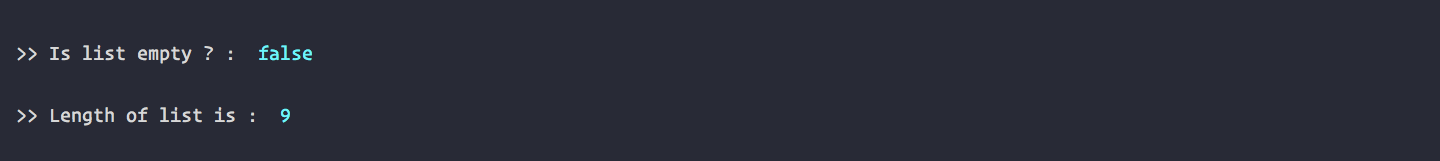IsEmpty & Length Operation

#### 🔅 Traverse

`traverse( fn ) {   let current = this.head;   while( current !== null ) {    fn(current)    current = current.next;   }   return true;}`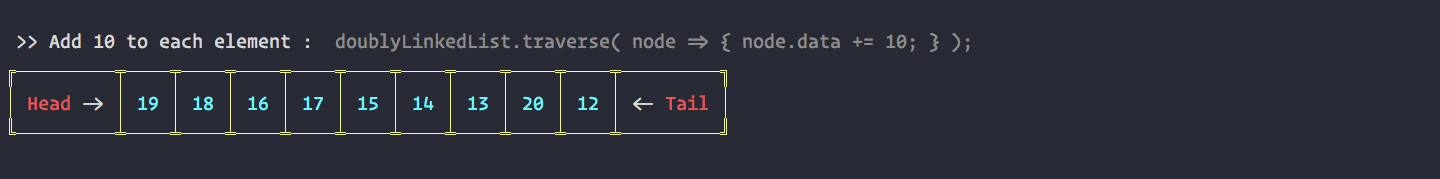Traverse Operation

#### 🔅 TraverseReverse

`traverseReverse( fn ) {  let current = this.tail;  while( current !== null ) {   fn(current)   current = current.prev;  }  return true;}`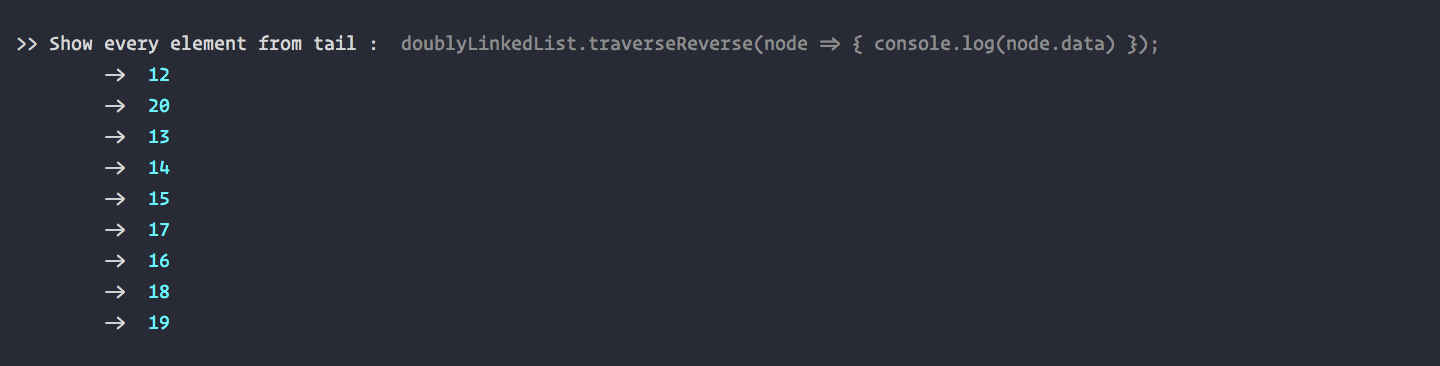TraverseReverse Operation

#### 🔅 Search

`search( item ) {  let current = this.head;  let counter = 0;`
`  while( current ) {    if( current.data == item ) {     return counter    }    current = current.next    counter++  }  return false;}`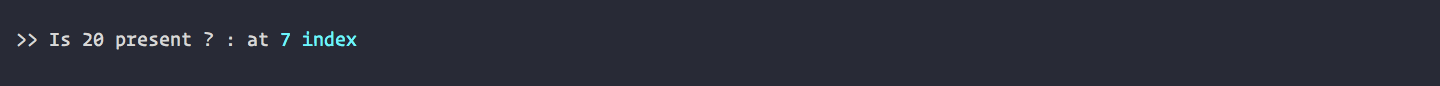Search Opeartion

### Practice

1. GeekForGeeks Double Linked List
2. HackerRank Linked Lists

### One more important thing..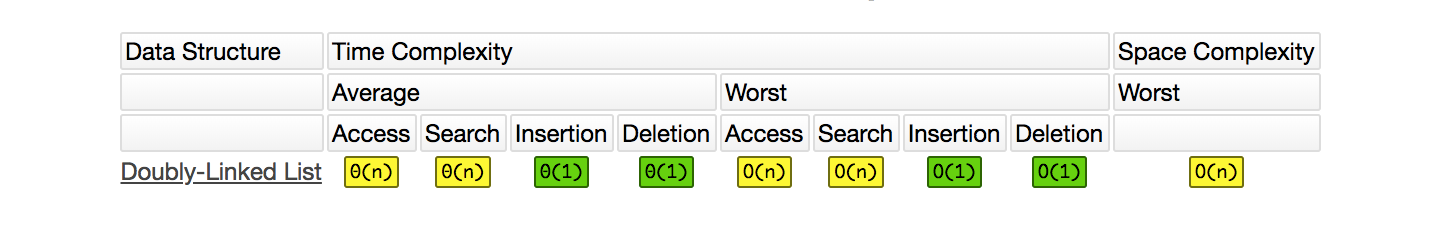For details — http://bigocheatsheet.com/

### About this post

This post is the second instalment to its series “DS with JS”. There will be more in this series. Next will be on next Thursday Morning. Stay tuned!

### That’s It

#### Happy Coding !!

🎧 No music today… I lost something very important today…

If you like this article, please give it some claps 👏 and share it! If you do not like this post or have any type of questions regarding anything that i mentioned in this post. Feel free to ask me. Just post an issue in my “Ask Me Anything” by clicking here.

For more like this, follow me on Medium or Twitter. To ask a Question visit this link. More about me on my website.